Try out our new practice tests completely free!# Algebra and Trigonometry

Mathematics

## Quiz 1 : Fundamental Concepts of AlgebraStudy FlashcardsLooking for Calculus Homework Help?

## Quiz 1 :Fundamental Concepts of AlgebraThe two given numbers are coordinates of points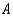and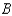, respectively, on a coordinate line. Express the indicated statement as an inequality involving the absolute value symbol.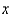,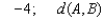is at least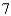Free
Multiple Choice

B

Tags
Choose question tagFactor the polynomial.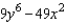Free
Multiple Choice

B

Tags
Choose question tagExpress as a polynomial.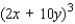Free
Multiple Choice

E

Tags
Choose question tagExpress as a polynomial.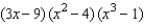Multiple Choice
Tags
Choose question tagRewrite the expression using a radical.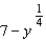Multiple Choice
Tags
Choose question tagFactor the polynomial.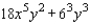Multiple Choice
Tags
Choose question tagSimplify the expression.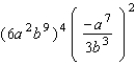Multiple Choice
Tags
Choose question tagExpress as a polynomial.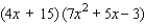Multiple Choice
Tags
Choose question tagSimplify the expression: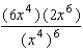Multiple Choice
Tags
Choose question tagApproximate the real-number expression to four decimal places.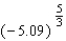Multiple Choice
Tags
Choose question tagFactor the polynomial.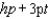Multiple Choice
Tags
Choose question tagExpress the following statement as an inequality. a is not less than 2
Multiple Choice
Tags
Choose question tagReplace the symbol "?" to make the resulting statement true for all real numbers : q, b, and c, whenever the expressions are defined.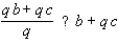Multiple Choice
Tags
Choose question tagApproximate the real-number expression to four decimal places.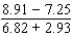Multiple Choice
Tags
Choose question tagIn astronomy, distances to stars are measured in light years. One light year is the distance a ray of light travels in one year. If the speed of light is approximately 186,000 miles per second, estimate the number of miles in two light years.
Multiple Choice
Tags
Choose question tagSimplify the expression.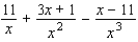Multiple Choice
Tags
Choose question tagSimplify the expression.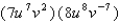Multiple Choice
Tags
Choose question tagWrite the expression as a simplified rational number.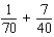Multiple Choice
Tags
Choose question tagApproximate the real-number expression to four decimal places.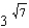Multiple ChoiceSimplify the expression.